Question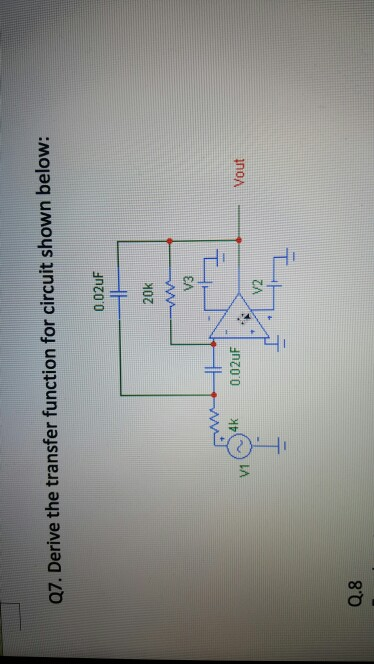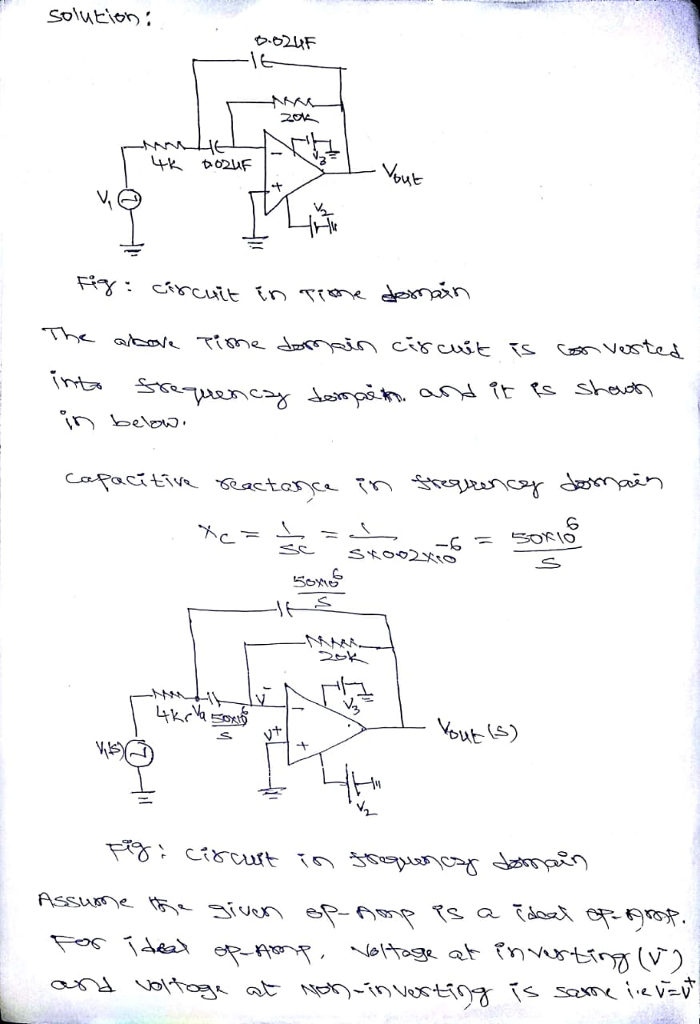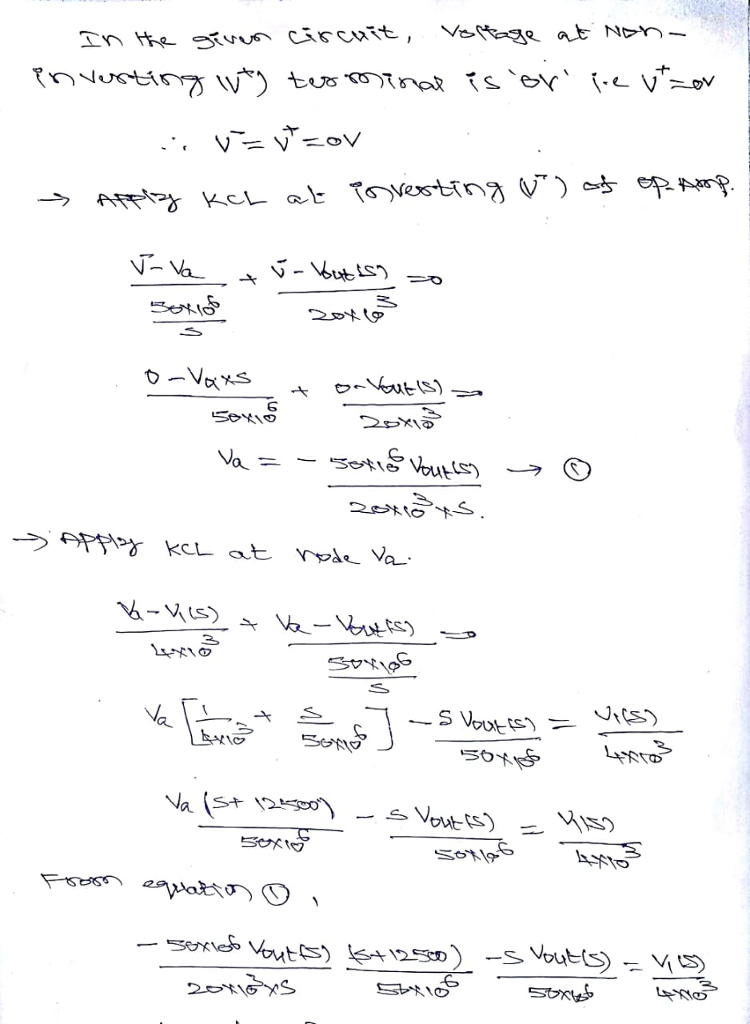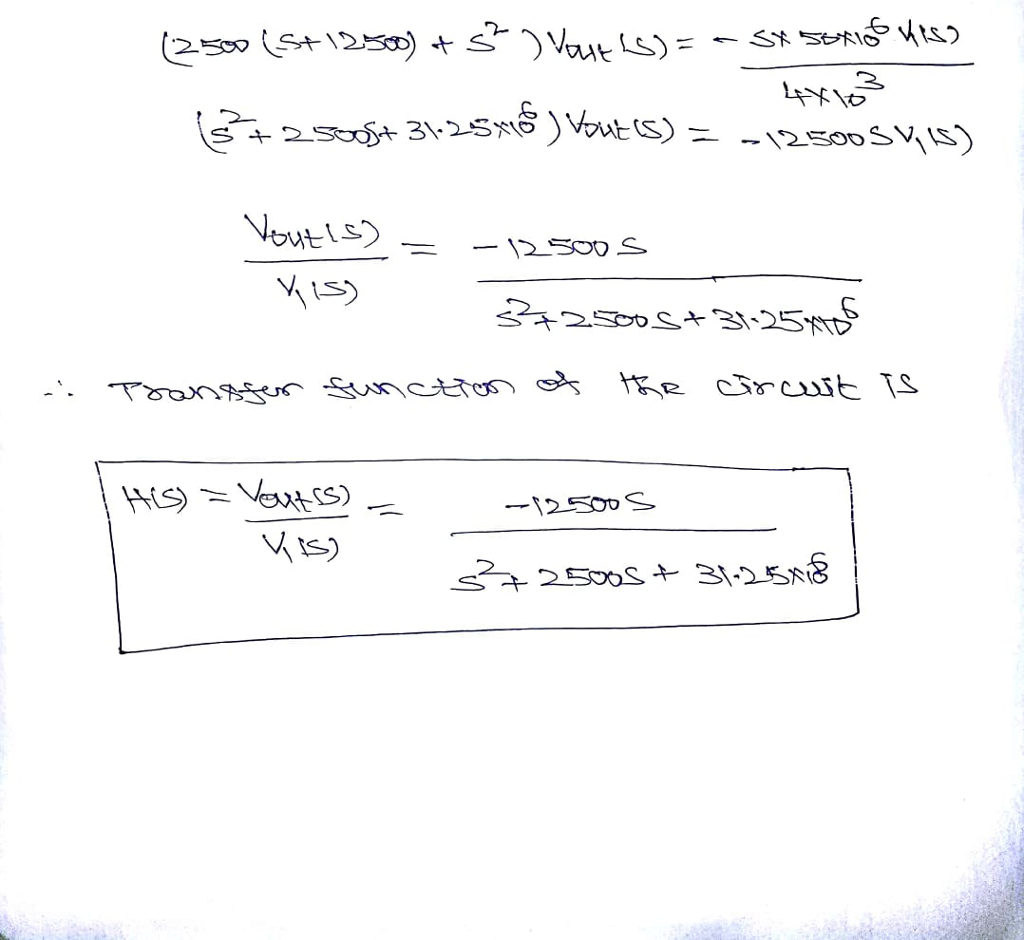#### Earn Coins

Coins can be redeemed for fabulous gifts.

Similar Homework Help Questions
• ### Q11 (20pts.): For the circuit shown below Derive the transfer function, H(s) assuming the OPAMP's transfer...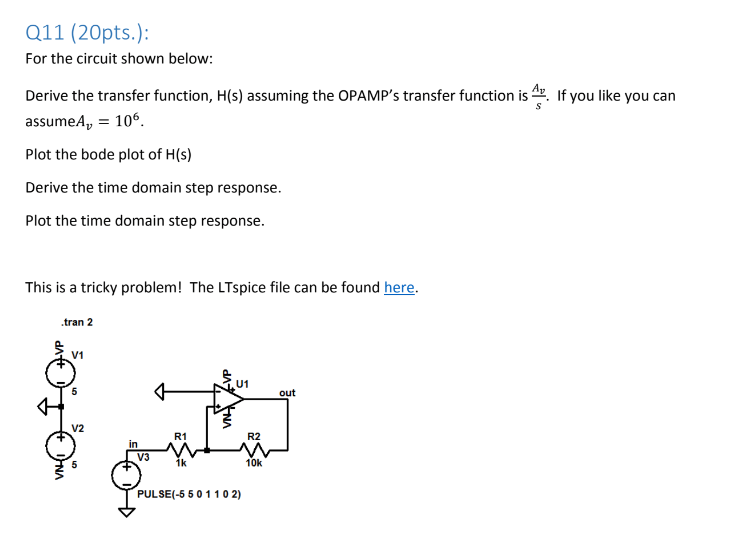Q11 (20pts.): For the circuit shown below Derive the transfer function, H(s) assuming the OPAMP's transfer function is. If you like you can assumeA 106 Plot the bode plot of H(s) Derive the time domain step response. Plot the time domain step response This is a tricky problem! The LTspice file can be found here. .tran 2 V1 U1 out V2 R1 R2 in V3 1k 10k PULSE(-5 50 1102)

• ### For the circuit shown below (1) Please derive an expression for the transfer function H(jco)- Vout/Vin....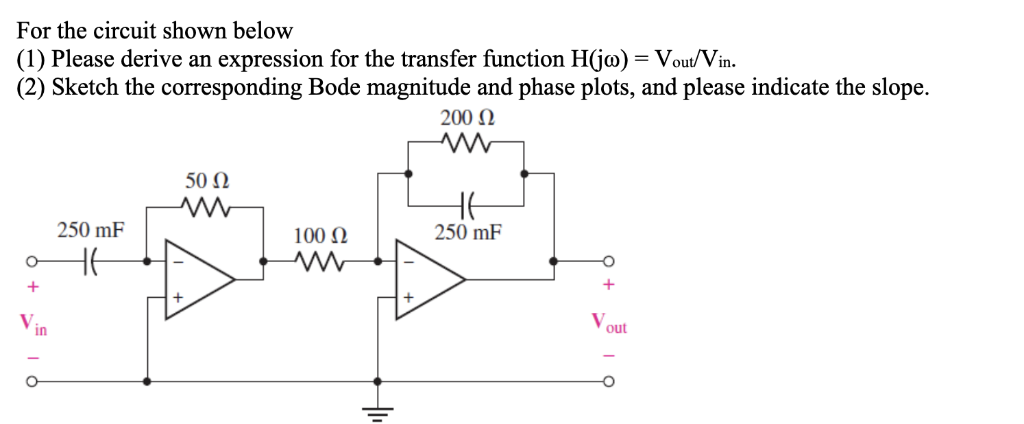For the circuit shown below (1) Please derive an expression for the transfer function H(jco)- Vout/Vin. (2) Sketch the corresponding Bode magnitude and phase plots, and please indicate the slope. 50Ω 250 mF 100 Ω 250 mF in out

• ### The transfer function of the circuit below is provided in Figure A. Assuming the diodes to...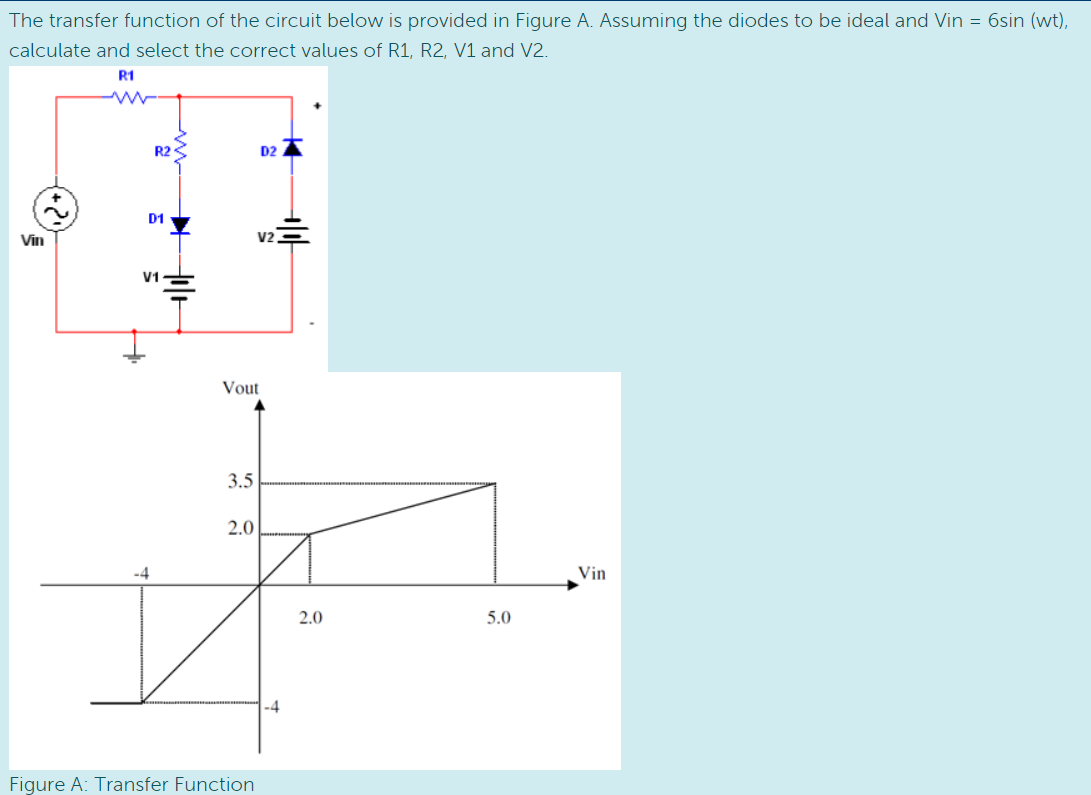The transfer function of the circuit below is provided in Figure A. Assuming the diodes to be ideal and Vin = 6sin (wt), calculate and select the correct values of R1, R2, V1 and V2. - R2Z 2 Vout 3.5 5.0 Figure A: Transfer Function

• ### QUESTION #2 PLEASE 1. Derive the transfer function for the circuit shown below. Plot H(s) versus...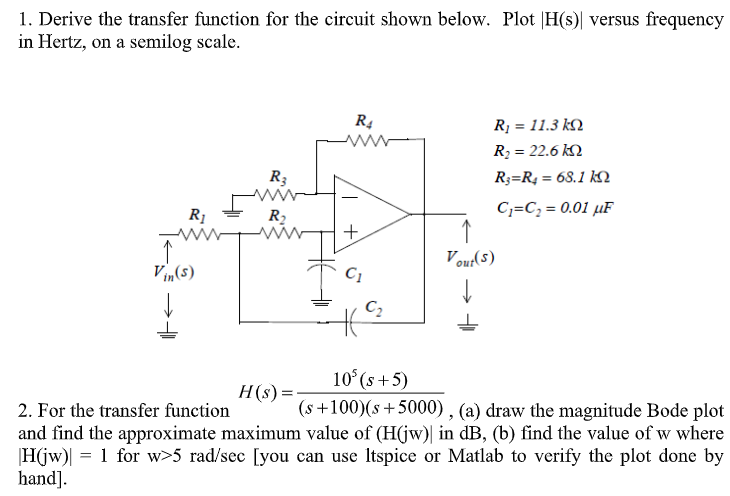QUESTION #2 PLEASE 1. Derive the transfer function for the circuit shown below. Plot H(s) versus frequency in Hertz, on a semilog scale. Ri 11.3 k Ri 22.6 k R R = 68.1 kN R3 C C 0.01 uF R2 Vout(s) Vin(s) C2 10 (s+5) H(s) = (s+100)(s5000) , (a) draw the magnitude Bode plot 2. For the transfer function and find the approximate maximum value of (H(jw) in dB, (b) find the value of w where 1 for w>5...

• ### Vout(s) Vin(s) For the circuit shown below, derive the conditions that will make the trasnfer fun...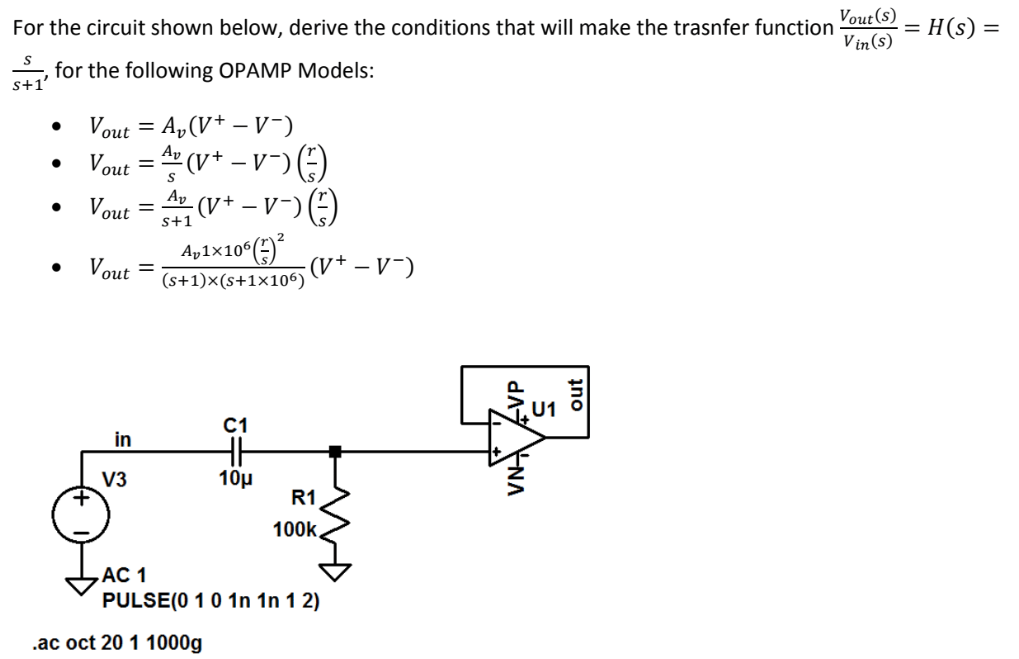Please show all work. Vout(s) Vin(s) For the circuit shown below, derive the conditions that will make the trasnfer function--= H(s) = , for the following OPAMP Models: S+1 out s+1 2 Av1x106 out (s+1)x (s+1x106) C1 in V3 10μ R1 100k AC 1 PULSE(0 10 1n 1n 1 2) .ac oct 20 1 1000g Vout(s) Vin(s) For the circuit shown below, derive the conditions that will make the trasnfer function--= H(s) = , for the following OPAMP Models: S+1...

• ### 10. Derive the transfer function for the circuit below, where Vs is the input, and vo...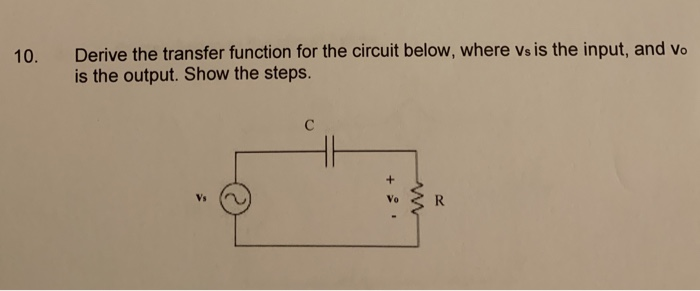10. Derive the transfer function for the circuit below, where Vs is the input, and vo is the output. Show the steps.

• ### Please ,determine V1, V2 and V3 for the circuit below (assume ideal diodes)!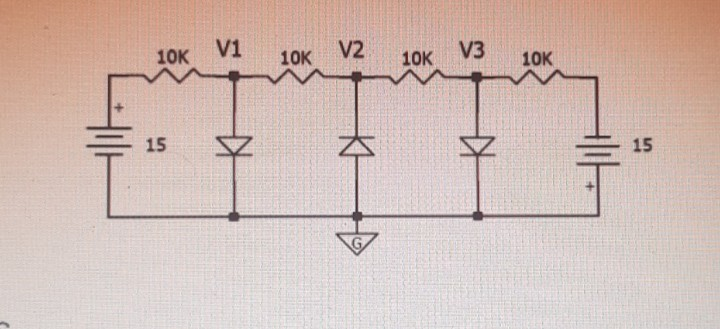Determine V1, V2 and V3 for the circuit below (assume ideal diodes).

• ### 1. Derive the transfer function for the circuit shown below. Plot FH(s) versus frequency in Hertz,...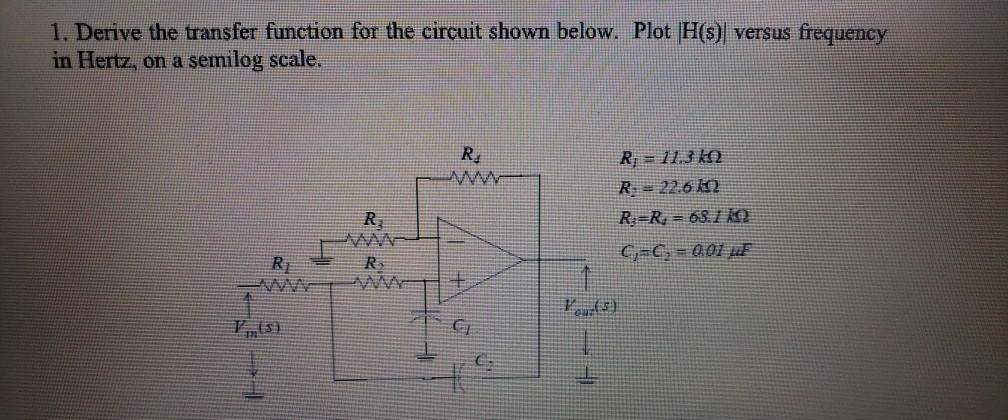1. Derive the transfer function for the circuit shown below. Plot FH(s) versus frequency in Hertz, on a semilog scale. Re R 113 k R 22.6 12 R R 63.12 Rj F)

• ### pls help R1 R3 R2 w w V14 V3+ V2+ Consider the circuit shown below, where...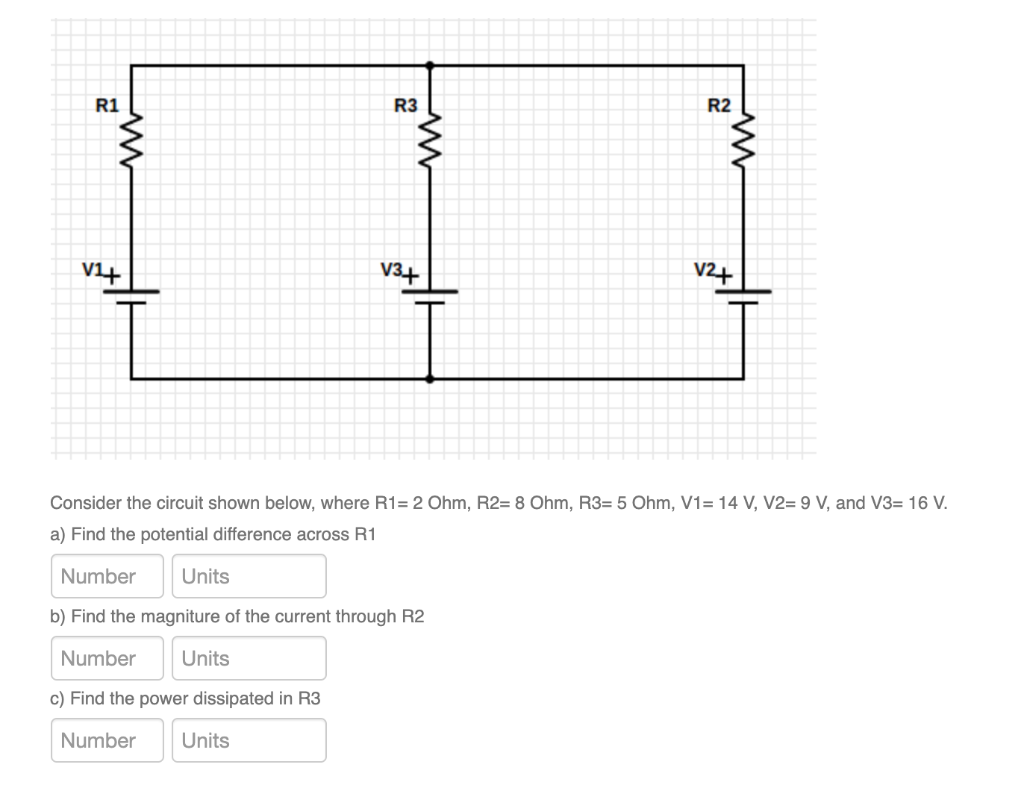pls help R1 R3 R2 w w V14 V3+ V2+ Consider the circuit shown below, where R1= 2 Ohm, R2= 8 Ohm, R3= 5 Ohm, V1= 14 V, V2= 9 V, and V3= 16 V. a) Find the potential difference across R1 Number Units b) Find the magniture of the current through R2 Number Units c) Find the power dissipated in R3 Number Units

• ### Problem 1: (Time Response) Derive the transfer function (s) of the electrical circuit shown below. Then...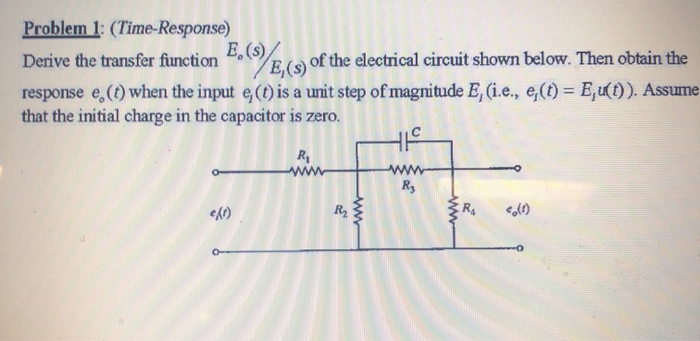Problem 1: (Time Response) Derive the transfer function (s) of the electrical circuit shown below. Then obtain the response e (0) when the input (1) is a unit step of magnitude E, (i.e., e(t) = E, (t)). Assume that the initial charge in the capacitor is zero. e/o)